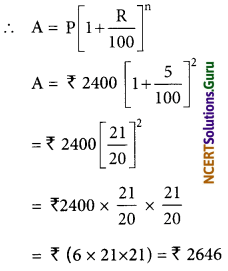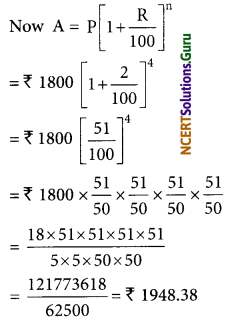# NCERT Solutions for Class 8 Maths Chapter 8 Comparing Quantities InText Questions

These NCERT Solutions for Class 8 Maths Chapter 8 Comparing Quantities InText Questions and Answers are prepared by our highly skilled subject experts.

## NCERT Solutions for Class 8 Maths Chapter 8 Comparing Quantities InText Questions

Try These (Page No. 119)

Question 1.
In a primary school, the parents were asked about the number of hours they spend per day in helping their children to do homework.
There were 90 parents who helped for $$\frac{1}{2}$$ hour to 1$$\frac{1}{2}$$ hours. The distribution of parents according to the time for which, they said they helped is given in the following figure: 20% helped for more than 1$$\frac{1}{2}$$ hours per day; 30% helped for $$\frac{1}{2}$$ hour to 1$$\frac{1}{2}$$ hours; 50% did not help at all.
(i) How many parents were surveyed?
(ii) How many said that they did not help?
(iii) How many said that they helped for man than 1$$\frac{1}{2}$$ hours?Solution:
(i) Since, 30% of total surveyed parents helped their children for “$$\frac{1}{2}$$ hours to 1$$\frac{1}{2}$$ hours”. And 90 parents helped their children for “$$\frac{1}{2}$$ hours to 1$$\frac{1}{2}$$ hours”.
30% [ surveyed parents] = 90
or $$\frac{30}{100}$$ × [surveyed parents] = 90
or surveyed parents = 90 × 100/30
= 3 × 100
= 300(ii) Since, 50% of surveyed parents did not help their children.
Number of parents who did not help = 50% of surveyed parents
= 50% of 300
= 50/100 × 300
= 150

(iii) Since, 20% of surveyed parents help their children for more than 1$$\frac{1}{2}$$ hours, i.e. 20% of surveyed parents help for more than 1$$\frac{1}{2}$$ hours.
Number of parents who helped for more than 1$$\frac{1}{2}$$ hours = 20% of 300
= 20/100 × 300
= 20 × 3
= 60
Note: ‘of’ means multiplication

Try These (Page No. 121)

Question 2.
A shop gives a 20% discount. What would the sale price of each of these be?
(a) A dress marked at ₹ 120
(b) A pair of shoes marked at ₹ 750
(c) A bag marked at ₹ 250
Solution:
(a) Marked price of the dress = ₹ 120
Discount rate = 20%
Discount = 20% of ₹ 120
= ₹ $$\frac{20}{100}$$ × 120
= ₹ 2 × 12
= ₹ 24
Sale price of the dress = [Marked Price] – [Discount]
= ₹ 120 – ₹ 24
= ₹ 96

(b) Marked price of the pair of shoes = ₹ 750
Discount rate = 20%
Discount = 20% of ₹ 750
= ₹ $$\frac{20}{100}$$ × 750
= ₹ 2 × 75
= ₹ 150
Now, Sale price of the pair of shoes = [Marked Price] – [Discount]
= ₹ 750 – ₹ 150
= ₹ 600

(c) Marked price of the bag = ₹ 250
Discount rate = 20%
Discount = 20% of ₹ 250
= ₹ $$\frac{20}{100}$$ × 250
= ₹ 2 × 25
= ₹ 50
Sale price of the bag = [Marked price] – [ Discount]
= ₹ 250 – ₹ 50
= ₹ 200Question 3.
A table marked at ₹ 15,000 is available for ₹ 14,000. Find the discount given and the discount percent.
Solution:
The marked price of the table = ₹ 15000
Sale price of the table = ₹ 14400
Discount = [Marked Price] – [Sale price]
= ₹ 15000 – ₹ 14400
= ₹ 600
Discount percent = Discount/Marked Price × 100

Question 4.
An almirah is sold at ₹ 5,225 after allowing a discount of 5%. Find its marked price.
Solution:
Sale price of the almirah = ₹ 5255
Discount rate = 5%
Since, Discount = 5% of marked price
Marked Price – Discount = Sale price
or [Marked Price] – $$\frac{5}{100}$$ × [Marked Price] = Sale price
or [Marked Price] × $$\frac{95}{100}$$ = ₹ 5255
or [Marked Price] = ₹ 5255 × $$\frac{95}{100}$$ = ₹ 5500

Try These (Page No. 123)

Question 5.
Find selling price (SP) if a profit of 5% is made on
(a) a cycle of ₹ 700 with ₹ 50 as overhead charges.
(b) a lawnmower bought at ₹ 1150 with ₹ 50 as transportation charges.
(c) a fan bought for ₹ 560 and expenses of ₹ 40 made on its repairs.
Solution:
(a) Total cost price = ₹ 700 + ₹ 50 (overhead expenses) = ₹ 750
profit = 5% of ₹ 750
= ₹ $$\frac{5}{100}$$ × ₹ 780
= ₹ 5 × $$\frac{15}{100}$$
= ₹ $$\frac{75}{100}$$
= ₹ 37.50
Now, SP = CP + profit
= ₹ 750 + ₹ 37.50
= ₹ 787.50(b) Total cost price = ₹ 1150 + ₹ 50 (Overhead expenses) = ₹ 1200
Profit = 5% of 1200
= $$\frac{5}{100}$$ × ₹ 1200
= ₹ 5 × 12
= ₹ 60
Now, SP = CP + Profit
= ₹ 1200 + ₹ 60
= ₹ 1260

(c) Total cost price = ₹ 560 + ₹ 40 (Overhead expenses) = ₹ 600
Profit = 5% of ₹ 600 = $$\frac{5}{100}$$ × ₹ 600
= ₹ 5 × 6
= ₹ 30
Now, SP = CP + profit
= ₹ 600 + ₹ 30
= ₹ 630

Try These (Page No. 123)

Question 6.
A shopkeeper bought two TV sets at ₹ 10,000 each. He sold one at a profit of 10% and the other at a loss of 10%. Find whether he made an overall profit or loss.
Solution:
(a) Marked price of the dress = ₹ 120
Discount rate = 20%
Discount = 20% of ₹ 120
= ₹ $$\frac{20}{100}$$ × 120
= ₹ 2 × 12
= ₹ 24
Sale price of the dress = [Marked Price] – [Discount]
= ₹ 120 – ₹ 24
= ₹ 96

(b) Marked price of the pair of shoes = ₹ 750
Discount rate = 20%
Discount = 20% of ₹ 750
= ₹ $$\frac{20}{100}$$ × 750
= ₹ 2 × 75
= ₹ 150
Now, Sale price of the pair of shoes = [Marked Price] – [Discount]
= ₹ 750 – ₹ 150
= ₹ 600(c) Marked price of the bag = ₹ 250
Discount rate = 20%
Discount = 20% of ₹ 250
= ₹ $$\frac{20}{100}$$ × 250
= ₹ 2 × 25
= ₹ 50
Sale price of the bag = [Marked price] – [Discount]
= ₹ 250 – ₹ 50
= ₹ 200

Try These (Page No. 126)

Question 7.
Find the interest and amount to be paid on ₹ 15000 at 5% per annum after 2 years.
Solution:
Here P = ₹ 15000, R% = 5%, T = 2 years
Simple Interest = $$\frac{\text { PRT }}{100}$$
= $$\frac{15000 \times 5 \times 2}{100}$$
= ₹ 1500
Amount = ₹ 15000 + ₹ 1500 = ₹ 16,500

Try These (Page No. 129)

Question 8.
Find Cl on a sum of ₹ 8000 for 2 years at 5% per annum compounded annually.
Solution:
We have
P = ₹ 8000, R = 55 p.a., T = 2 years
∴ $$A=P\left[1+\frac{R}{100}\right]^{n}$$
= ₹ 8000 $$\left[1+\frac{5}{100}\right]^{2}$$
= ₹ $$8000\left[\frac{21}{20}\right]^{2}$$
= ₹ $$8000 \times \frac{21}{20} \times \frac{21}{20}$$
= ₹ (20 × 21 × 21)
= ₹ 8820
Now, compound interest = A – P
= ₹ 8820 – ₹ 8000
= ₹ 820Try These (Page No. 130)

Question 9.
Find the time period and rate for each.
1. A sum taken for 1$$\frac{1}{2}$$ years at 8% per annum is compounded half-yearly.
2. A sum taken for 2 years at 4% per annum compounded half-yearly.
Solution:
1. We have interest rate 8% per annum 1$$\frac{1}{2}$$ year.
It is compounded half-yearly.
Time period (n) = 2(1$$\frac{1}{2}$$) = 3 half yearly
Rate (R) = $$\frac{1}{2}$$ (8%) = 4% per half yearly.

2. We have an interest rate of 4% per annum for the year
Time period (n) = 2(2) = 4 half-yearly
Rate (R) = $$\frac{1}{2}$$ (4%) = 2% per half yearly.

Try These (Page No. 131)

Question 10.
Find the amount to be paid
1. At the end of 2 years on ₹ 2,400 at 5% per annum compounded annually.
2. At the end of 1 year on ₹ 1,800 at 8% per annum compounded quarterly.
Solution:
1. We have:
P = ₹ 2400, R = 5% p.a., T = 2 years
∴ Interest is compounded annually i.e, n = 22. Here, interest compounded quarterly.
R = 8%, p.a. = $$\frac{8}{4}$$, i.e., 2% per quarter
T = 1 year = 4 × 1, i.e., 4 quarters on
n = 4Try These (Page No. 133)

Question 11.
A machinery worth ₹ 10,500 depreciated by 5%. Find its value after one year?
Solution:
Here, P = 10, 500, R = 5% p.a.
T = 1 year, n = 1
∴ A = $$\mathrm{P}\left[1+\frac{5}{100}\right]^{1}$$
[∴ Depreciation is there, r = -5%]
= ₹ $$10500\left[1+\frac{5}{100}\right]^{1}$$
= ₹ 10500 × $$\frac{19}{20}$$
= ₹ 525 × 19
= ₹ 9975
Thus, machinery value after 1 year = ₹ 9975
Note: For depreciation, we use the formula as A = $$\mathrm{P}\left[1-\frac{\mathrm{R}}{100}\right]^{\mathrm{n}}$$Question 12.
Find the population of a city after 2 years, which is at present 12 lakh, if the rate of increase is 4%.
Solution:
Present population, P = 12 lakh
Rate of increase, R = 4% p.a.
Time, T = 2 years
n = 2
Population after 2 years = $$\mathrm{P}\left[1+\frac{\mathrm{R}}{100}\right]^{\mathrm{n}}$$
= 12 lakh $$\left[1+\frac{4}{100}\right]^{2}$$
= 12 lakh $$\left[\frac{26}{25}\right]^{2}$$
= 12,00, 000 × $$\frac{26}{25} \times \frac{26}{25}$$
= 1920 × 26 × 26
= 12,97,920
Thus, the population of the town will be 12,97,920 after 2 years.

error: Content is protected !!